# For the circuit shown in the figure above: Obtain an expression for H() Vo/Vi in standard form Generate spectral plots for the magnitude and phase of H (w), given that R-100Ω, L-0.1 mH, and C-1 μF De...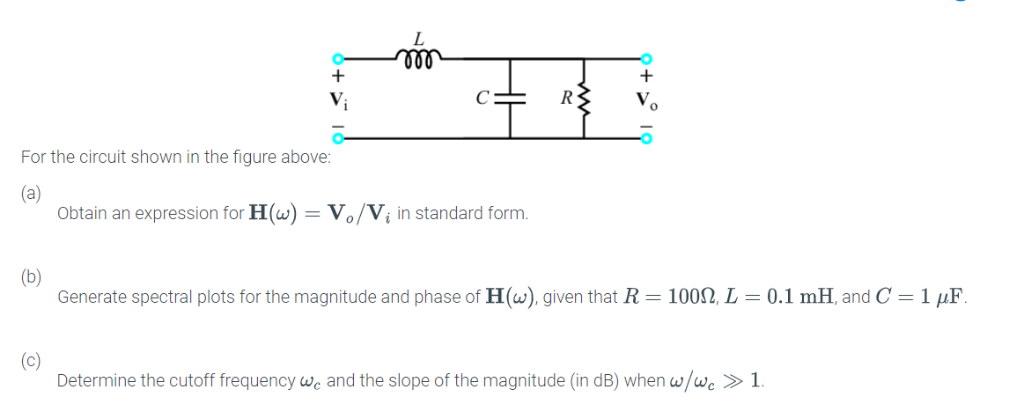For the circuit shown in the figure above: Obtain an expression for H() Vo/Vi in standard form Generate spectral plots for the magnitude and phase of H (w), given that R-100Ω, L-0.1 mH, and C-1 μF Determine the cutoff frequency we and the slope of the magnitude (in dB) when wwc >1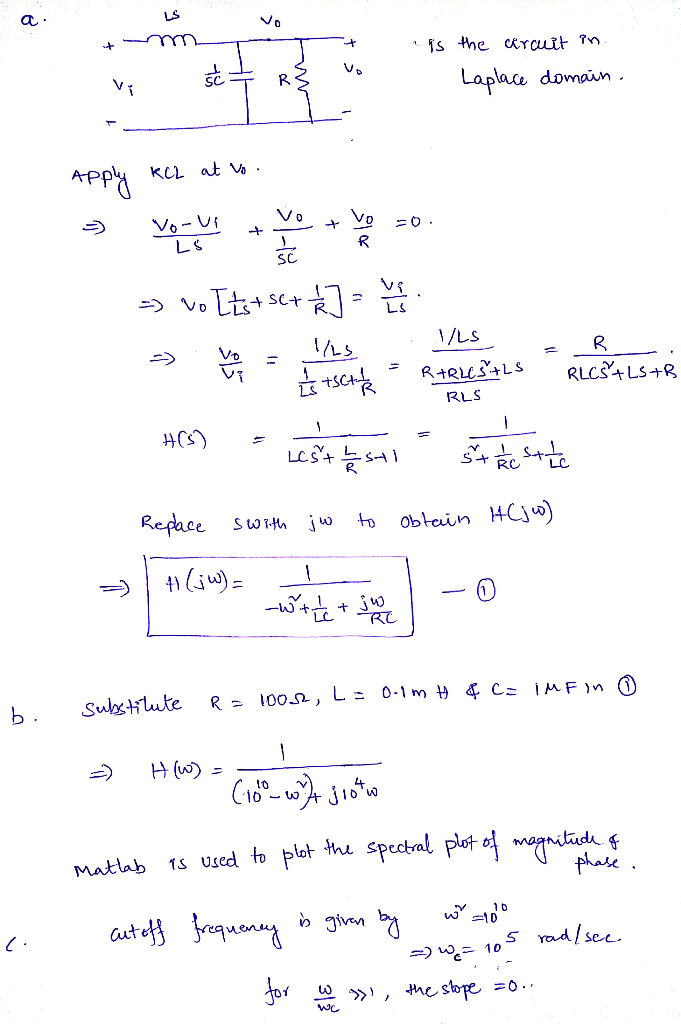MATLAB code is given below in bold letters.

r=100;l=0.1e-3;c=1e-6;
h=tf(1,[1 1/(r*c) 1/(l*c)])
figure;bode(h);grid on;

The magnitude and phase responses are plotted below.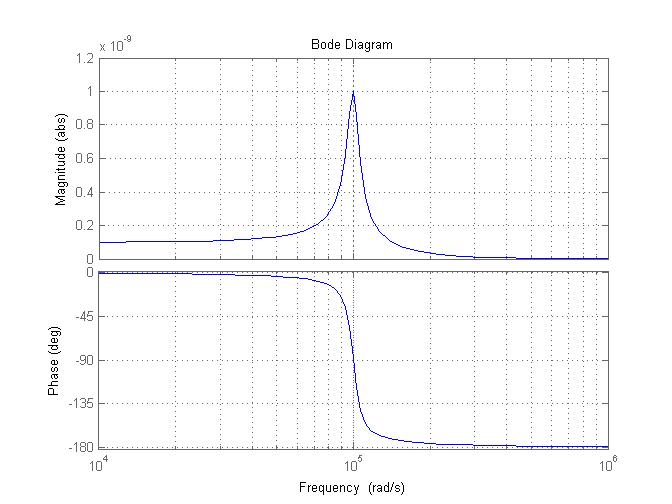##### Add Answer of: For the circuit shown in the figure above: Obtain an expression for H() Vo/Vi in standard form Generate spectral plots for the magnitude and phase of H (w), given that R-100Ω, L-0.1 mH, and C-1 μF De...
Similar Homework Help Questions
• ### Spectral plots of passive filter"a: Obtain an expression for H(ω) = Vo/Vi in standard form.b: Generate spectral plots for the magnitude and phase of H(ω), given that R1 = 1 Ω, R2 = 2 Ω, L1 = 1 mH, andL2 = 2 mH.c: Determine the cutoff frequency ωc and the slope of the magnitude (in dB) when ω/ωc << 1 and when ω/ωc>> 1."My question is, what is the standard-form expression for this transfer function, or how do I get it into standard form?...

• ### 21 Vi Z2 Vo Figure 1 1. Ref: Figure 1. Let Z1 L (an inductor), Z2 - R (a resistor). Vi Calculate the magnitude and phase of the transfer function H(w) Figure 1 T 2. Repeat #1 with L = 100 mH, R 1kΩ....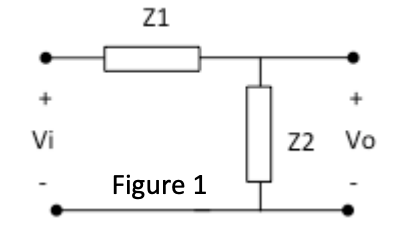21 Vi Z2 Vo Figure 1 1. Ref: Figure 1. Let Z1 L (an inductor), Z2 - R (a resistor). Vi Calculate the magnitude and phase of the transfer function H(w) Figure 1 T 2. Repeat #1 with L = 100 mH, R 1kΩ. a) Plot the frequency response in dB* on a both on a linear scale and then a log scale from ω-1 to 100,000,000 rad/sec with points every decade (1 b) 1,000 etc). 10 100 Plot the...

• ### 2. H(s)Vo/v, for the circuit shown in Figure P9-12. 1 ΜΩ Figure P9-12 2. H(s)Vo/v, for the circuit shown in Figure P9-12. 1 ΜΩ Figure P9-12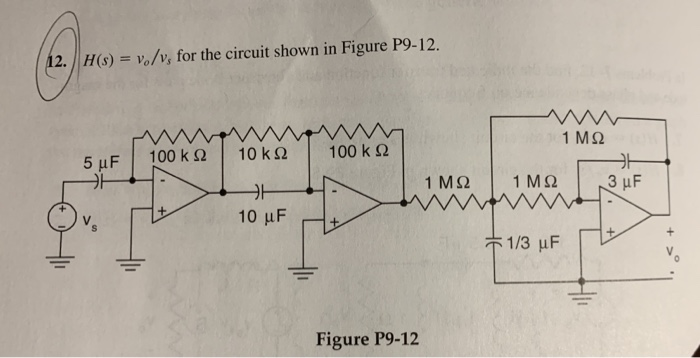2. H(s)Vo/v, for the circuit shown in Figure P9-12. 1 ΜΩ Figure P9-12 2. H(s)Vo/v, for the circuit shown in Figure P9-12. 1 ΜΩ Figure P9-12

• ### For the circuit shown in figure 7, find the node voltages Vi. V2 and Va nalysis 12 (4 V 2Ω 4 A Fig.7 h. For the circuit...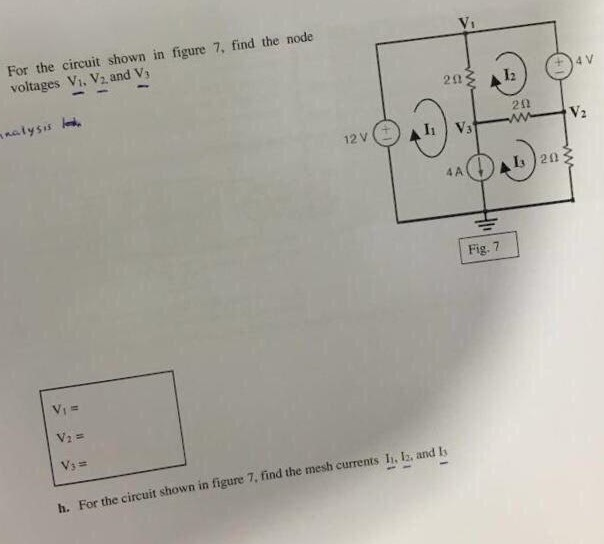For the circuit shown in figure 7, find the node voltages Vi. V2 and Va nalysis 12 (4 V 2Ω 4 A Fig.7 h. For the circuit shown in figure 7, find the mesh currents I, la, and Is For the circuit shown in figure 7, find the node voltages Vi. V2 and Va nalysis 12 (4 V 2Ω 4 A Fig.7 h. For the circuit shown in figure 7, find the mesh currents I, la, and Is

• ### 2. In the circuit shown in Figure 2, let C=0.5 F L=1H, R-312. Find the voltage vo(t) for t>0 L volt) Figure 2 2. In the circuit shown in Figure 2, let C=0.5 F L=1H, R-312. Find the voltage vo...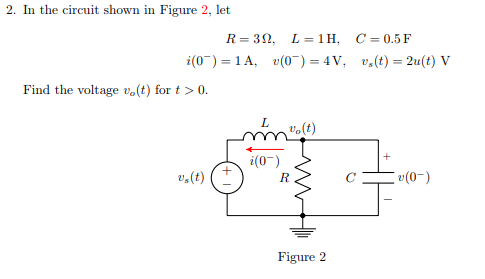2. In the circuit shown in Figure 2, let C=0.5 F L=1H, R-312. Find the voltage vo(t) for t>0 L volt) Figure 2 2. In the circuit shown in Figure 2, let C=0.5 F L=1H, R-312. Find the voltage vo(t) for t>0 L volt) Figure 2

• ### 2. Given the following circuit RL Do where C = inF, L = 1 mH and R1 = į00kf2. (a) Derive the expression for the tra...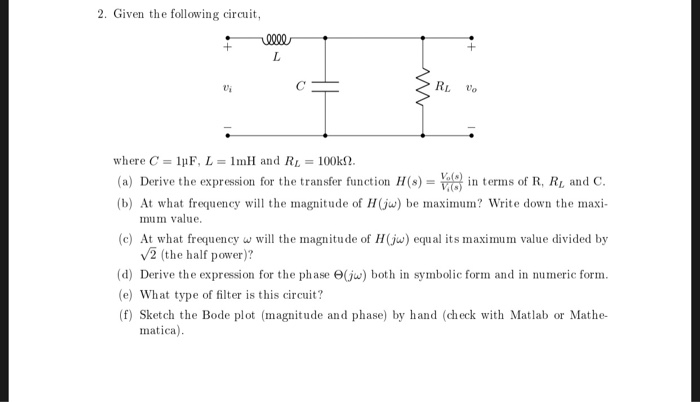2. Given the following circuit RL Do where C = inF, L = 1 mH and R1 = į00kf2. (a) Derive the expression for the transfer functionH(s)0 in erms of R, Ri and C. (b) At what frequency will the magnitude of Hju) be maximum? Write down the maxi- mum value (c) At what frequency w will the magnitude of H(jw) equal its maximum value divided by V2 (the half power)? (d) Derive the expression for the phase e(ju) both...

• ### Circuit Analysis in the s-Domain 15.3. The initial voltage across the capacitor in the circuit shown in Figure P15.3 is v(0) 1 V, and the initial current through the inductor is i(0)0 mA Find th...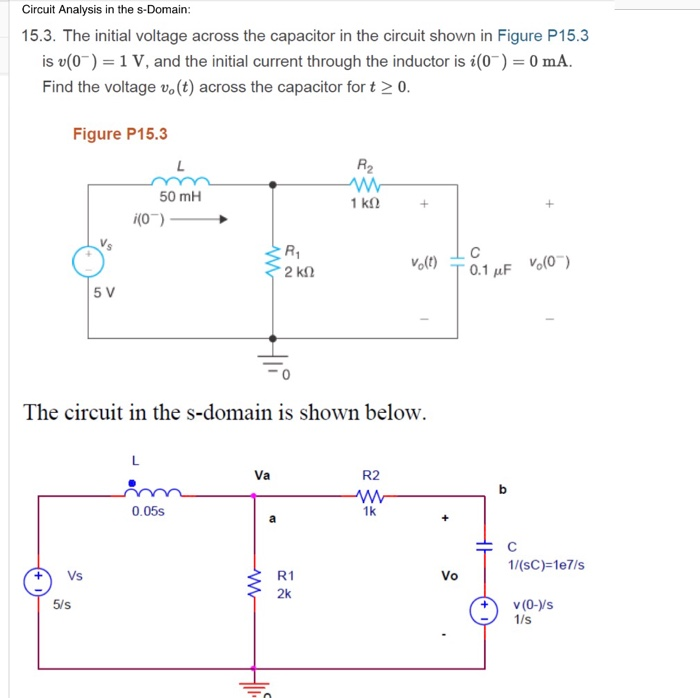Circuit Analysis in the s-Domain 15.3. The initial voltage across the capacitor in the circuit shown in Figure P15.3 is v(0) 1 V, and the initial current through the inductor is i(0)0 mA Find the voltage vo (t) across the capacitor for t 2 0 Figure P15.3 50 mH 1 kS2 V. Volt) T 0.1 μF The circuit in the s-domain is shown below. R2 Va 1k 0.05s 1/(sC)-1e7/s Vo R1 2k V (0-ys 5/s 1/s 1 format long; 2...

• ### 752) See Figure 752. D-4. The Bode gain and phase plots for a RC circuit are phase (deg), and frequency B. Also find the other shown in the fig. Determine gain (dB), (rad/sec) for the points la...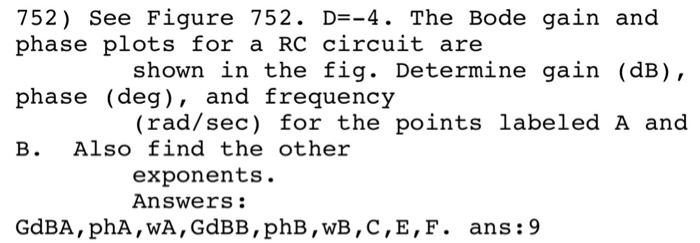752) See Figure 752. D-4. The Bode gain and phase plots for a RC circuit are phase (deg), and frequency B. Also find the other shown in the fig. Determine gain (dB), (rad/sec) for the points labeled A and exponents. Answers: GdBA, phA, wA, GdBB, phB,wB,C, E,F. ans:9 Bode Gain and Phase Plots Gain in dB 6 -10 12.64 10 Frequency in rad/sec 10 10 Phase in deg 765 40 10 F 4764 Frequency in rad/sec Figure 752 10 10...

• ### For the circuit shown in the figure, (a) find the current in resistor R1 and (b) find Va-Vi-Let E...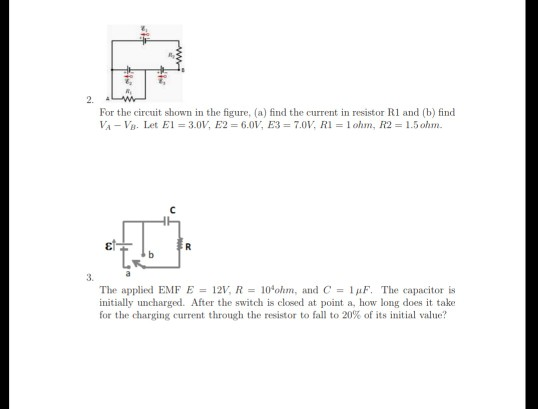For the circuit shown in the figure, (a) find the current in resistor R1 and (b) find Va-Vi-Let El = 3.0V, E2 = 6.0V, E3 = 7.01, Ri = l ohm, R2 = 1.5ohm. εί 3. The applied EMF E = 121, R 10Ohm, and C = 1 μF. The capacitor is initially uncharged. After the switch is closed at point a, how long does it take for the dunging current through the resistor to fall to 20% of its...

• ### Pre-Laboratory Task 4: Derive an expression for the magnitude of the transfer function, H(Go)Vout(jo)/Wn(j, and the phase of the transfer function LH (ja) for the LCR circuit in Figure 4. Plot H(ja)l...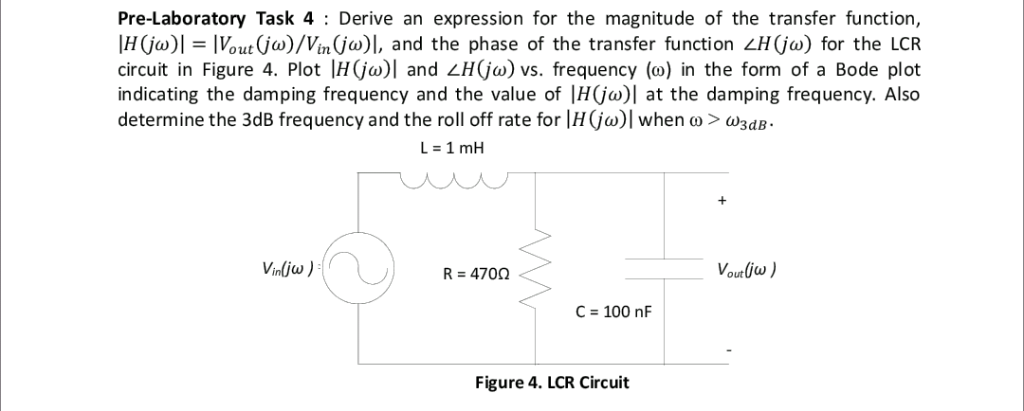Pre-Laboratory Task 4: Derive an expression for the magnitude of the transfer function, H(Go)Vout(jo)/Wn(j, and the phase of the transfer function LH (ja) for the LCR circuit in Figure 4. Plot H(ja)l and H(jo) vs. frequency (o) in the form of a Bode plot indicating the damping frequency and the value of |H(jo)| at the damping frequency. Also determine the 3dB frequency and the roll off rate for Ir(ja)1 when ω > ω3dB. Vounlius R 470Ω C 100 nF Figure...

Need Online Homework Help?